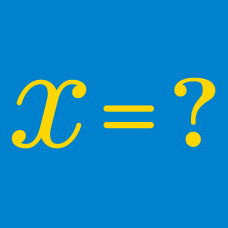Algebra

# Algebra Warmups: Level 2 Challenges

Evaluate the following expression:

$123456789^{2} - (123456788 \times 123456790).$

If you use a calculator whose precision is not strong enough to answer this question, then you will answer this problem incorrectly.

$\large\color{#20A900} 6^{\color{#3D99F6} 6} + \color{#20A900} 6^{\color{#3D99F6} 6} + \color{#20A900} 6^{\color{#3D99F6} 6} + \color{#20A900} 6^{\color{#3D99F6} 6} + \color{#20A900} 6^{\color{#3D99F6} 6} + \color{#20A900} 6^{\color{#3D99F6} 6} = \color{#20A900}6^ {\color{#624F41}a}$

If $\color{#624F41} a$ satisfies the equation above, what is the value of $\color{#624F41} a$?

What is the value of

$\large \sqrt{1\text{\%}}\, ?$

$\large \frac { 1 }{ { 2 }^{ 3 } } +\frac { 1 }{ { 2 }^{ 6 } } +\frac { 1 }{ { 2 }^{ 9 } } + \cdots = \, ?$

If 7 cows need 7 days to eat 7 grass fields, how many days would it take for 5 cows to eat 5 grass fields?

×